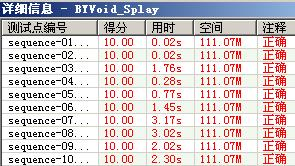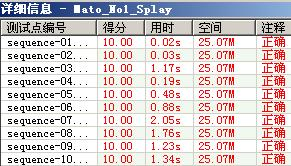【原题见这里

Splay Tree之所以可以当线段树用，是因为它可以支持一个序列，然后用“左端前趋伸展到根，右端后继伸展到根的右子结点，取根的右子结点的左子结点”这种伸展方法，对一个序列中的一整段进行整体操作。由于要防止出现前趋或后继不存在的情况，需要在这个序列的两端加入两个边界结点，要求其值不能影响到结点各种记载信息的维护（多取0、∞或-∞）。这两个边界结点在树中永远存在，不会被删除。

（1）结点的引用：

（2）标记：

（3）自底向上维护的信息：

（4）对连续插入的结点建树：

（5）回收空间：

【2012年1月16日更新】

#include <iostream>
#include
<stdio.h>
using namespace std;
#define re(i, n) for (int i=0; i<n; i++)
#define re1(i, n) for (int i=1; i<=n; i++)
const int MAXN = 500002, NOSM = -2000, INF = ~0U >> 2;
struct node {

int v, c, p, sz, sum, lmax, rmax, midmax, sm;

bool rev, d;
} T[MAXN
+ 1];
int root, Q[MAXN + 1], front, rear, a[MAXN], len, res;
int max(int SS0, int SS1)
{

return SS0 >= SS1 ? SS0 : SS1;
}
int max(int SS0, int SS1, int SS2)
{

int M0 = SS0 >= SS1 ? SS0 : SS1; return M0 >= SS2 ? M0 : SS2;
}
void newnode(int n, int _v)
{
T[n].v
= T[n].sum = T[n].lmax = T[n].rmax = T[n].midmax = _v; T[n].c[0= T[n].c[1= 0; T[n].sz = 1; T[n].sm = NOSM; T[n].rev = 0;
}
void sc(int _p, int _c, bool _d)
{
T[_p].c[_d]
= _c; T[_c].p = _p; T[_c].d = _d;
}
void sm_opr(int x, int SM)
{
T[x].sum
= T[x].sz * SM;

if (SM > 0) T[x].lmax = T[x].rmax = T[x].midmax = T[x].sum; else T[x].lmax = T[x].rmax = T[x].midmax = SM;
}
void rev_opr(int x)
{

int c0 = T[x].c, c1 = T[x].c; sc(x, c0, 1); sc(x, c1, 0);

int tmp = T[x].lmax; T[x].lmax = T[x].rmax; T[x].rmax = tmp;
}
void dm(int x)
{

int SM0 = T[x].sm;

if (SM0 != NOSM) {
T[x].v
= T[T[x].c].sm = T[T[x].c].sm = SM0; T[x].sm = NOSM;
sm_opr(T[x].c[
0], SM0); sm_opr(T[x].c, SM0);
}

if (T[x].rev) {
T[T[x].c[
0]].rev = !T[T[x].c].rev; T[T[x].c].rev = !T[T[x].c].rev; T[x].rev = 0;
rev_opr(T[x].c[
0]); rev_opr(T[x].c);
}
}
void upd(int x)
{

int c0 = T[x].c, c1 = T[x].c;
T[x].sz
= T[c0].sz + T[c1].sz + 1;
T[x].sum
= T[c0].sum + T[c1].sum + T[x].v;
T[x].lmax
= max(T[c0].lmax, T[c0].sum + T[x].v + max(T[c1].lmax, 0));
T[x].rmax
= max(T[c1].rmax, max(T[c0].rmax, 0+ T[x].v + T[c1].sum);
T[x].midmax
= max(T[c0].midmax, T[c1].midmax, max(T[c0].rmax, 0+ T[x].v + max(T[c1].lmax, 0));
}
void rot(int x)
{

int y = T[x].p; bool d = T[x].d;

if (y == root) {root = x; T[root].p = 0;} else sc(T[y].p, x, T[y].d);
sc(y, T[x].c[
!d], d); sc(x, y, !d); upd(y);
}
void splay(int x, int r)
{

int p; while ((p = T[x].p) != r) if (T[p].p == r) rot(x); else if (T[x].d == T[p].d) {rot(p); rot(x);} else {rot(x); rot(x);} upd(x);
}
int Find_Kth(int K)
{

int i = root, S0;

while (i) {
dm(i); S0
= T[T[i].c].sz + 1;

if (K == S0) breakelse if (K < S0) i = T[i].c; else {K -= S0; i = T[i].c;}
}

return i;
}
int mkt(int l, int r)
{

if (l > r) return 0;

int n0 = Q[front], mid = l + r >> 1if (front == MAXN) front = 1else front++;
newnode(n0, a[mid]);
int l_r = mkt(l, mid - 1), r_r = mkt(mid + 1, r);
sc(n0, l_r,
0); sc(n0, r_r, 1); upd(n0); return n0;
}
void ins(int pos)
{

int P0 = Find_Kth(pos); splay(P0, 0); int P1 = Find_Kth(pos + 1); splay(P1, root); sc(P1, mkt(0, len - 1), 0); upd(P1); upd(P0);
}
void era(int x)
{

if (!x) return;

if (rear == MAXN) rear = 1else rear++; Q[rear] = x;
era(T[x].c[
0]); era(T[x].c);
}
void del(int l, int r)
{

int P0 = Find_Kth(l - 1); splay(P0, 0); int P1 = Find_Kth(r + 1); splay(P1, root);

int root0 = T[P1].c; sc(P1, 00); upd(P1); upd(P0); era(root0);
}
void mksame(int l, int r, int x)
{

int P0 = Find_Kth(l - 1); splay(P0, 0); int P1 = Find_Kth(r + 1); splay(P1, root);

int n = T[P1].c; T[n].sm = x; sm_opr(n, x); upd(P1); upd(P0);
}
void reve(int l, int r)
{

int P0 = Find_Kth(l - 1); splay(P0, 0); int P1 = Find_Kth(r + 1); splay(P1, root);

int n = T[P1].c; T[n].rev = !T[n].rev; rev_opr(n); upd(P1); upd(P0);
}
int get_sum(int l, int r)
{

int P0 = Find_Kth(l - 1); splay(P0, 0); int P1 = Find_Kth(r + 1); splay(P1, root);

int n = T[P1].c; return T[n].sum;
}
int max_sum()
{

return T[root].midmax;
}
void prepare()
{
T[
0].sz = T.sum = T.lmax = T.rmax = T.midmax = 0;
front
= 3; rear = MAXN; re1(i, MAXN) Q[i] = i;
newnode(
1-INF); newnode(2-INF); sc(121); root = 1; T[root].p = 0;
}
int main()
{
freopen(
"sequence.in""r", stdin);
freopen(
"sequence.out""w", stdout);
prepare();

int m, l, r, x;
scanf(
"%d%d"&len, &m); char ch = getchar(), str;
re(i, len) scanf(
"%d"&a[i]); ins(1);
re(i, m) {
scanf(
"%s", str);

if (!strcmp(str, "INSERT")) {scanf("%d%d"&l, &len); re(i, len) scanf("%d"&a[i]); ins(++l);}

if (!strcmp(str, "DELETE")) {scanf("%d%d"&l, &r); r += l++; del(l, r);}

if (!strcmp(str, "MAKE-SAME")) {scanf("%d%d%d"&l, &r, &x); r += l++; mksame(l, r, x);}

if (!strcmp(str, "REVERSE")) {scanf("%d%d"&l, &r); r += l++; reve(l, r);}

if (!strcmp(str, "GET-SUM")) {scanf("%d%d"&l, &r); r += l++; printf("%d\n", get_sum(l, r));}

if (!strcmp(str, "MAX-SUM")) printf("%d\n", max_sum());
ch
= getchar();
}
fclose(stdin); fclose(stdout);

return 0;
}

BYVoid神犇的：【相关论文】

【感谢】
JZP神犇（提供论文）
BYVoid神犇（提供标程）

 只有注册用户登录后才能发表评论。 【推荐】超50万行VC++源码: 大型组态工控、电力仿真CAD与GIS源码库 相关文章: